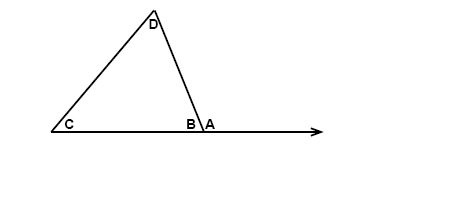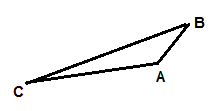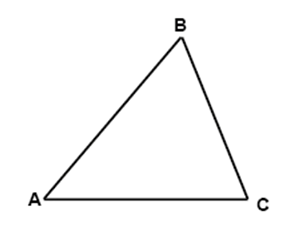# Inequalities

The exterior angle of a triangle is always greater than either of its corresponding interior angles:$\\ m\angle A> m\angle C\\ m\angle A> m\angle D\\$

Two important things to remember is that if one side of a triangle is longer than another side (compare BC and AB in the triangle below BC > AB) then the angle opposite of the longer side is always greater than the angle opposite (A > B) of the shorter side. Likewise if one angle is greater than another angle (A > B) then the side opposite of the greater angle is always longer than the side opposite the lesser angle (BC > AB).The sum of the lengths of any two sides in a triangle is always greater than the length of the third side:$AB+BC>AC$

$AC+AB>BC$

$BC+AC>AB$

## Video lesson

List the sides of the triangle in the order from shortest to longest.